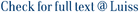The paper develops a theory of biases in decision making. Discovering a strategy for solving a game is a complex problem that may be solved by decomposition; a player decomposing a problem into many simple sub-problems may easily identify the optimal solution to each sub-problem: however it is shown that even though all partial solutions are optimal, the solution to the global problem may be largely sub-optimal. The conditions under which a decomposition process gives rise to a sub-optimal solution are explored, and it is shown that the sub-optimalities ultimately originate from the process of categorization that governs the creation of a decomposition pattern. Decisions based on a strategy discovered by decomposition are therefore frequently biased . The persistence of biased behaviours, observed in many experiments, is explained by showing the stability of different and non optimal representations of the same problem. An application to a simplified version of Rubik cube is finally developed.

Decomposition patterns in problem solving / Egidi, Massimo. - (2007), pp. 15-46.

### Decomposition patterns in problem solving

#### Abstract

The paper develops a theory of biases in decision making. Discovering a strategy for solving a game is a complex problem that may be solved by decomposition; a player decomposing a problem into many simple sub-problems may easily identify the optimal solution to each sub-problem: however it is shown that even though all partial solutions are optimal, the solution to the global problem may be largely sub-optimal. The conditions under which a decomposition process gives rise to a sub-optimal solution are explored, and it is shown that the sub-optimalities ultimately originate from the process of categorization that governs the creation of a decomposition pattern. Decisions based on a strategy discovered by decomposition are therefore frequently biased . The persistence of biased behaviours, observed in many experiments, is explained by showing the stability of different and non optimal representations of the same problem. An application to a simplified version of Rubik cube is finally developed.
##### Scheda breve Scheda completa Scheda completa (DC)0444522425
9780444522429
Decomposition patterns in problem solving / Egidi, Massimo. - (2007), pp. 15-46.
File in questo prodotto:
File
PaperEgidi_4696Pubblicato.pdf

Solo gestori archivio

Tipologia: Documento in Post-print
Licenza: DRM non definito
Dimensione 989.36 kB
Utilizza questo identificativo per citare o creare un link a questo documento: `http://hdl.handle.net/11385/4696`
•ND
•ND